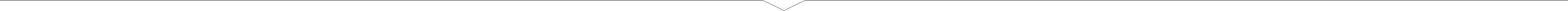0 | \$0.00

### BMR & TDEE Calculator

The basal metabolic rate (BMR) and calorie calculator is a excellent tool for working out how many calories your body needs on a daily basis depending on the amount and intensity of your exercise regime.

### Instructions:

This BMR tool works on a proven formula and is very accurate. The calculator uses two formulas to calculate your body's daily calorie requirements.

Basal Metabolic Rate (BMR) Formula
The basal metabolic rate formula takes several variables like height, weight, age and gender to calculate your calorie needs as if you did not do any exercise.

Harris Benedict Formula
The Harris Benedict Formula takes the number produced by the BMR formula and multiplies it depending on your activity level.

The only factor it omits is lean body mass and thus the ratio of muscle-to-fat a body has. Remember, leaner bodies need more calories than less leaner ones. Therefore, this equation will be very accurate in all but the very muscular (will underestimate calorie needs) and the very overweight (will over-estimate calorie needs).